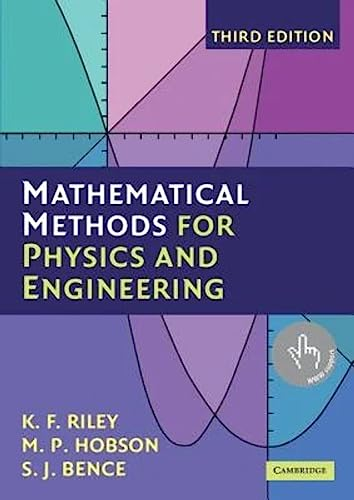# Mathematical Methods for Physics and Engineering by K. F. Riley (University of Cambridge)

Out of Stock
\$59.99
inc. GST
This highly acclaimed undergraduate textbook teaches all the mathematics for undergraduate courses in the physical sciences. Containing over 800 exercises, half come with hints and answers and, in a separate manual, complete worked solutions. The remaining exercises are intended for unaided homework; full solutions are available to instructors.
Only 0 left

## Mathematical Methods for Physics and Engineering Summary

### Mathematical Methods for Physics and Engineering: A Comprehensive Guide by K. F. Riley (University of Cambridge)

The third edition of this highly acclaimed undergraduate textbook is suitable for teaching all the mathematics for an undergraduate course in any of the physical sciences. As well as lucid descriptions of all the topics and many worked examples, it contains over 800 exercises. New stand-alone chapters give a systematic account of the 'special functions' of physical science, cover an extended range of practical applications of complex variables, and give an introduction to quantum operators. Further tabulations, of relevance in statistics and numerical integration, have been added. In this edition, half of the exercises are provided with hints and answers and, in a separate manual available to both students and their teachers, complete worked solutions. The remaining exercises have no hints, answers or worked solutions and can be used for unaided homework; full solutions are available to instructors on a password-protected web site, www.cambridge.org/9780521679718.

### Why buy from World of BooksOur excellent value books literally don't cost the earthFree delivery in AustraliaEvery used book bought is one saved from landfill

## Mathematical Methods for Physics and Engineering Reviews

From reviews of previous editions: '...a great scientific textbook. It is a tour de force ... to write mathematical sections that are both complete and at an appropriate academic level. The authors have clearly succeeded in this challenge, making this a remarkable pedagogical book ... The choice of exercises is excellent and possibly the best feature of the book. In summary, this textbook is a great reference at undergraduate levels, particularly for those who like to teach or learn using lots of examples and exercises.' R. Botet, European Journal of Physics
'... the book provides scientists who need to use the tool of mathematics for practical purposes with a single, comprehensive book. I recommend this book not only to students in physics and engineering sciences, but also to students in other fields of natural sciences.' P. Steward, Optik
'... suitable as a textbook for undergraduate use ... this is a book that in view of its content and its modest softcover price, will find its way on to many bookshelves.' Nigel Steele, The Times Higher Education Supplement
'Riley et al. has clear, thorough and straightforward explanations of the subjects treated. It rigorously adopts a three-stage approach throughout the book: first a heuristic, intuitive introduction, then a formal treatment, and finally one or two examples. This consistent presentation, the layout, and the print quality make the book most attractive ... and value for money. It contains a thousand pages, there are plenty of exercises with each chapter.' J. M. Thijssen, European Journal of Physics
This is a valuable book with great potential use in present-day university physics courses. Furthermore, the book will be useful for graduate too, and researchers will find it useful for looking up material which they have forgotten since their undergraduate days.' J. M. Thijssen, European Journal of Physics
'This textbook is a well-written, modern, comprehensive, and complete collection of topics in mathematical methods ranging from a review of differential and integral calculus to group and representation theory, probability, the calculus of variations, and tensors.' Science Books and Films
'This is a very comprehensive textbook suitable for most students enrolling on undergraduate degree courses in engineering. It contains 31 stand-alone chapters of mathematical methods which enable the students to understand the principles of the basic mathematical techniques and the authors have produced a clear, thorough and straightforward explanation of each subject. ... finding a single textbook which covers the engineering student's need throughout their entire course is by no means an easy task. I believe the authors have achieved it ... complete fully worked solutions ... which I think is a useful asset for both students and lecturers.' Civil Engineering
' ... this highly acclaimed undergraduate textbook is suitable for teaching all the mathematics ever likely to be needed for an undergraduate course in any of the physical sciences. As well as lucid descriptions of all the topics covered and many worked examples, it contains more than 800 exercises.' L'enseignement mathematique

Prefaces; 1. Preliminary algebra; 2. Preliminary calculus; 3. Complex numbers and hyperbolic functions; 4. Series and limits; 5. Partial differentiation; 6. Multiple integrals; 7. Vector algebra; 8. Matrices and vector spaces; 9. Normal modes; 10. Vector calculus; 11. Line, surface and volume integrals; 12. Fourier series; 13. Integral transforms; 14. First-order ordinary differential equations; 15. Higher-order ordinary differential equations; 16. Series solutions of ordinary differential equations; 17. Eigenfunction methods for differential equations; 18. Special functions; 19. Quantum operators; 20. Partial differential equations: general and particular; 21. Partial differential equations: separation of variables; 22. Calculus of variations; 23. Integral equations; 24. Complex variables; 25. Application of complex variables; 26. Tensors; 27. Numerical methods; 28. Group theory; 29. Representation theory; 30. Probability; 31. Statistics; Index.

GOR003584369
Mathematical Methods for Physics and Engineering: A Comprehensive Guide by K. F. Riley (University of Cambridge)
K. F. Riley (University of Cambridge)
Used - Very Good
Paperback
Cambridge University Press
2006-03-13
1359
0521679710
9780521679718
N/A
Book picture is for illustrative purposes only, actual binding, cover or edition may vary.
This is a used book - there is no escaping the fact it has been read by someone else and it will show signs of wear and previous use. Overall we expect it to be in very good condition, but if you are not entirely satisfied please get in touch with us.